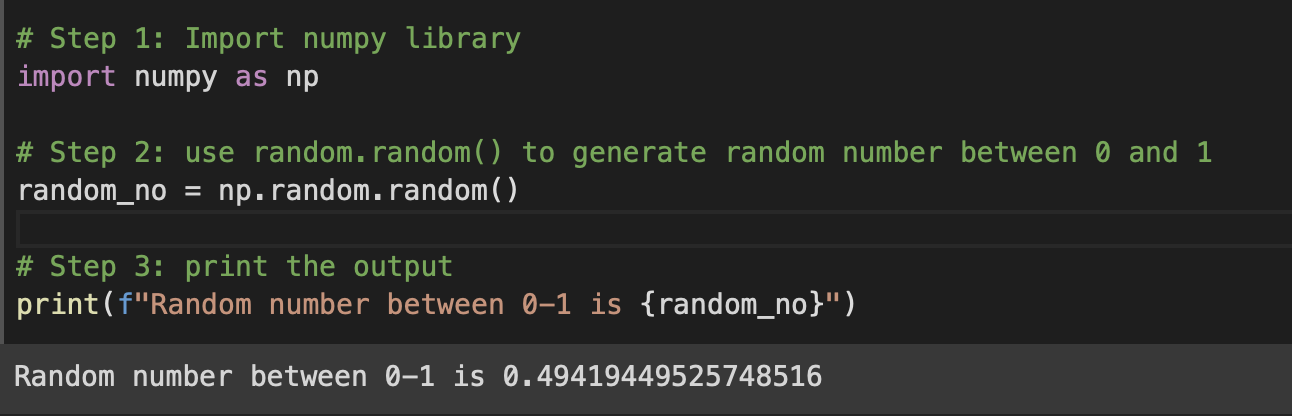# Python Program: Use NumPy to generate a random number between 0 and 1

To find a random number between 0 and 1 we will make use of the random.rand() function from the NumPy module.

## Code Example 1: random.rand(1)

``````# Step 1: Import numpy library
import numpy as np

# Step 2: Use random.rand function and get an array
random_no = np.random.rand(1)

# Step 3: Print the first element of the array
print("Random Number Between 0-1 is :", random_no)``````
Output:

0.5152378042391298

## Code Example 2: random.random()

``````#### use numpy to generate a random number between 0 and 1

# Step 1: Import numpy library
import numpy as np

# Step 2: use random.random() to generate random number between 0 and 1
random_no = np.random.random()

# Step 3: print the output
print(f"Random number between 0-1 is {random_no}")``````
Output:

Random number between 0-1 is 0.49419449525748516-

### Facing issues? Have Questions? Post them here! I am happy to answer!

Author Info:

Rakesh (He/Him) is a seasoned developer with over 10 years of experience in web and app development, and a deep knowledge of operating systems. Author of insightful How-To articles for Code2care.